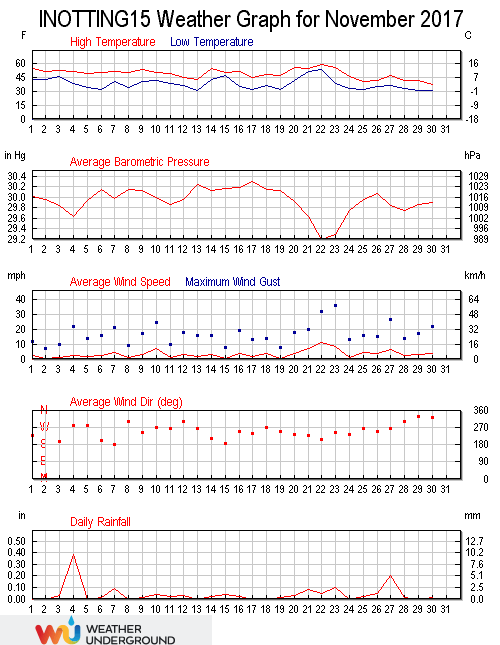November 2017 was cooler than normal. Winds were lighter than average and rainfall was well below normal.

The maximum temperature in the month was 14.5°C at 13:29 on the 22nd, 0.4°C below the average monthly maximum for November. The minimum temperature was -0.9°C at 05:05 on the 30th, 1.2°C above the average monthly minimum for the month.. This was the lowest temperature since -1.9°C was recorded on April 25th 2017. The first air frost of the autumn season occurred on the 13th when the temperature fell to -0.6°C. The monthly average temperature was 6.2°C, 0.6°C below the mean. The maximum temperature of 2.9°C, recorded at 14:29 on the 30th, was the lowest daily maximum temperature since February 12th 2017 when the maximum temperature only reached 2.7°C. The average daily maximum temperature was 9.2°C (0.3°C below the mean), and the average daily minimum temperature was 3.2°C (0.9°C below the mean). The warmest day was the 22nd with an average temperature of 13.2°C. The coldest day was the 30th with an average temperature of 0.9°C.

The highest dew point was 11.8°C recorded at 06:23 on the 22nd, 1.5°C below the November average. The lowest dew point was -3.9°C recorded at 08:04 on the 30th, 0.2°C below the November average low. The average dew point for the month was 4.4°C, 0.9°C below the November mean. The high wind chill was 13.4°C, recorded at 14:49 on the 22nd. The low wind chill was -3.5°C, recorded at 07:00 on the 30th. The average wind chill for the month was 5.4°C. There were 30.58 hours of sunshine in November with the sunniest day being the 8th when 4.22 hours was recorded.

Rainfall in November was 31.8 millimetres, well below the average monthly total of 60.2 millimetres. Rainfall was recorded on 21 days, with 2.0 millimetres of snow being recorded on the 30th. This was the first snowfall since February 11th 2017. The wettest day was the 4th when 10.0 millimetres of rainfall was recorded. The highest rain rate was 25.8 millimetres per hour recorded at 04:29 on the 23rd. There have been 408.0 millimetres of rain so far this year, 82% of the average by the end of November.

The highest barometric pressure was 1028.9 millibars at 10:14 on the 17th. The lowest barometric pressure was 978.8 millibars at 01:01 on the 23rd. The average pressure in the month was 1013.7 millibars. The highest humidity was 100% and the month average humidity was 88% equalling the lowest November average humidity. The lowest humidity was 61% at 13:56 on the 8th.

The highest wind gust in the month was 36 mph recorded at 14:06 on the 23rd. The average gust speed in the month was 5.2 mph, mean 6.7 mph. The average wind speed was 3.3 mph, mean 4.1 mph. The maximum wind speed was 18 mph at 14:16 on the 23rd, 4.6 mph below the mean. The total wind run was 2379.6 miles, with the predominate direction being 248°.

The maximum high solar radiation recorded at 11:53 on the 5th was 512 w/m². The average solar radiation for the month was 37 w/m². The highest U.V. Index was 0.7 set at 12:49 on the 11th.This site uses Akismet to reduce spam. Learn how your comment data is processed.